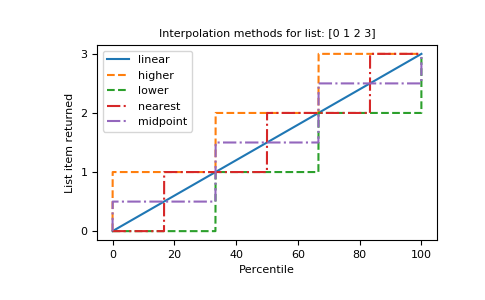# numpy.percentile¶

`numpy.``percentile`(a, q, axis=None, out=None, overwrite_input=False, interpolation='linear', keepdims=False)[source]

Compute the q-th percentile of the data along the specified axis.

Returns the q-th percentile(s) of the array elements.

Parameters: a : array_like Input array or object that can be converted to an array. q : array_like of float Percentile or sequence of percentiles to compute, which must be between 0 and 100 inclusive. axis : {int, tuple of int, None}, optional Axis or axes along which the percentiles are computed. The default is to compute the percentile(s) along a flattened version of the array. Changed in version 1.9.0: A tuple of axes is supported out : ndarray, optional Alternative output array in which to place the result. It must have the same shape and buffer length as the expected output, but the type (of the output) will be cast if necessary. overwrite_input : bool, optional If True, then allow the input array a to be modified by intermediate calculations, to save memory. In this case, the contents of the input a after this function completes is undefined. interpolation : {‘linear’, ‘lower’, ‘higher’, ‘midpoint’, ‘nearest’} This optional parameter specifies the interpolation method to use when the desired percentile lies between two data points `i < j`: ‘linear’: `i + (j - i) * fraction`, where `fraction` is the fractional part of the index surrounded by `i` and `j`. ‘lower’: `i`. ‘higher’: `j`. ‘nearest’: `i` or `j`, whichever is nearest. ‘midpoint’: `(i + j) / 2`. New in version 1.9.0. keepdims : bool, optional If this is set to True, the axes which are reduced are left in the result as dimensions with size one. With this option, the result will broadcast correctly against the original array a. New in version 1.9.0. percentile : scalar or ndarray If q is a single percentile and axis=None, then the result is a scalar. If multiple percentiles are given, first axis of the result corresponds to the percentiles. The other axes are the axes that remain after the reduction of a. If the input contains integers or floats smaller than `float64`, the output data-type is `float64`. Otherwise, the output data-type is the same as that of the input. If out is specified, that array is returned instead.

`mean`

`median`
equivalent to `percentile(..., 50)`

`nanpercentile`

`quantile`
equivalent to percentile, except with q in the range [0, 1].

Notes

Given a vector `V` of length `N`, the q-th percentile of `V` is the value `q/100` of the way from the minimum to the maximum in a sorted copy of `V`. The values and distances of the two nearest neighbors as well as the interpolation parameter will determine the percentile if the normalized ranking does not match the location of `q` exactly. This function is the same as the median if `q=50`, the same as the minimum if `q=0` and the same as the maximum if `q=100`.

Examples

```>>> a = np.array([[10, 7, 4], [3, 2, 1]])
>>> a
array([[10,  7,  4],
[ 3,  2,  1]])
>>> np.percentile(a, 50)
3.5
>>> np.percentile(a, 50, axis=0)
array([[ 6.5,  4.5,  2.5]])
>>> np.percentile(a, 50, axis=1)
array([ 7.,  2.])
>>> np.percentile(a, 50, axis=1, keepdims=True)
array([[ 7.],
[ 2.]])
```
```>>> m = np.percentile(a, 50, axis=0)
>>> out = np.zeros_like(m)
>>> np.percentile(a, 50, axis=0, out=out)
array([[ 6.5,  4.5,  2.5]])
>>> m
array([[ 6.5,  4.5,  2.5]])
```
```>>> b = a.copy()
>>> np.percentile(b, 50, axis=1, overwrite_input=True)
array([ 7.,  2.])
>>> assert not np.all(a == b)
```

The different types of interpolation can be visualized graphically:

```import matplotlib.pyplot as plt

a = np.arange(4)
p = np.linspace(0, 100, 6001)
ax = plt.gca()
lines = [
('linear', None),
('higher', '--'),
('lower', '--'),
('nearest', '-.'),
('midpoint', '-.'),
]
for interpolation, style in lines:
ax.plot(
p, np.percentile(a, p, interpolation=interpolation),
label=interpolation, linestyle=style)
ax.set(
title='Interpolation methods for list: ' + str(a),
xlabel='Percentile',
ylabel='List item returned',
yticks=a)
ax.legend()
plt.show()
```numpy.ptp

#### Next topic

numpy.nanpercentile